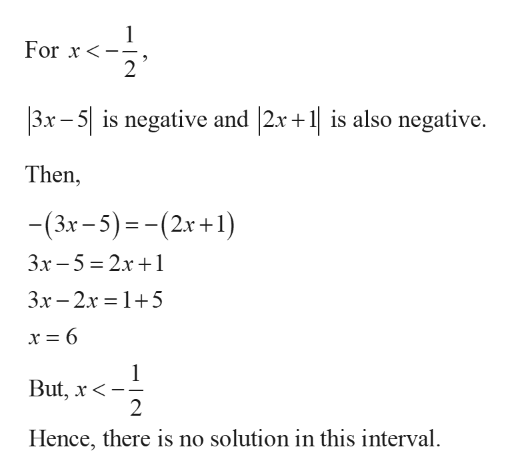# solve the equation or inequality. Write the solution set to the inequalities in interval notation.|3x − 5| = |2x + 1|

Question
22 views

solve the equation or inequality. Write the solution set to the inequalities in interval notation.

|3x − 5| = |2x + 1|

check_circle

Step 1

Given equation is,

|3x − 5| = |2x + 1|

Consider (3x – 5) = 0

3x = 5

x = 5/3

Consider (2x +1) = 0

2x = -1

x = -1/2

Step 2

For each absolutes form the below table,

Step 3

Hence,

...help_outlineImage TranscriptioncloseFor x <- 2 |3x - 5| is negative and |2x + 1| is also negative. Then, -(3x- 5) =-(2x+1) Зх -5 %3D 2х +1 Зх - 2х %3D 1+5 x = 6 1 But, x< Hence, there is no solution in this interval. fullscreen

### Want to see the full answer?

See Solution

#### Want to see this answer and more?

Solutions are written by subject experts who are available 24/7. Questions are typically answered within 1 hour.*

See Solution
*Response times may vary by subject and question.
Tagged in
MathAlgebra

### Other